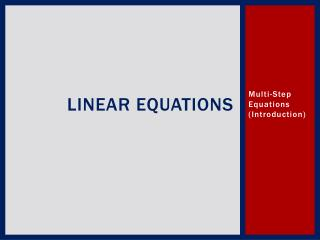DownloadDownload PresentationLinear Equations

# Linear Equations

Télécharger la présentation## Linear Equations

- - - - - - - - - - - - - - - - - - - - - - - - - - - E N D - - - - - - - - - - - - - - - - - - - - - - - - - - -
##### Presentation Transcript

1. Linear Equations Multi-Step Equations (Introduction)

2. Introduction to Solving Multi-Step Equations • How do gift wrap a present? • In your notes write down the steps for gift wrapping a present.

3. Introduction to Solving Multi-Step Equations • How do unwrap a present? • In your notes write down the steps for unwrapping a present. Pretend that you want to be able to use all your materials again. Meaning you don’t want to use scissors or tear anything.

4. Introduction to Solving Multi-Step Equations • PEMDAS or GEMDAS is similar to wrapping a present. First we do our parentheses or groupings, then exponents, next there’s multiplication or division, and finally addition or subtraction. • SADMEP or SADMEG is similar to unwrapping a present. We do all our previous steps in reverse. When we want to solve an equation we first do any addition or subtraction, then we do any multiplication or division that we can, next we work with the exponents and finally we work with our parentheses grouping.

5. Introduction to Solving Multi-Step Equations

6. Introduction to Solving Multi-Step Equations

7. Introduction to Solving Multi-Step Equations

8. Introduction to Solving Multi-Step Equations

9. Introduction to Solving Multi-Step Equations

10. Introduction to Solving Multi-Step Equations

11. Introduction to Solving Multi-Step Equations

12. Introduction to Solving Multi-Step Equations

13. Introduction to Solving Multi-Step Equations